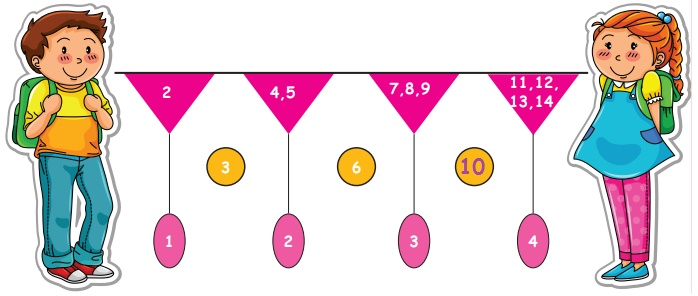Home | | Maths 5th Std | Relationship between consecutive square numbers and odd numbers

# Relationship between consecutive square numbers and odd numbers

To relate sequences of odd numbers between consecutive square numbers.

Patterns in Numbers

To relate sequences of odd numbers between consecutive square numbers.

Relationship between consecutive square numbers and odd numbers.

We have already learnt about the relationship between square numbers and triangular numbers.

Now we are going to know the relationship between consecutive square numbers and odd numbers.

When we add the consecutive odd numbers from 1, we will get the square numbers.Note: 1 is common number among square and triangular numbers.

Try this

a. 1 + 3 + 5 + 7 + 9 + 11 = 36 = 6 × 6 = 62

b. 1 + 3 + 5 + 7 + 9 + 11 + 13 = 49 = 7 × 7 = 72

c. 1 + 3 + 5 + 7 + 9 + 11 + 13 + 15 = 64 = 8 × 8 = 82

Find the missing number!Relationship between Square number and Triangle numbers

In mathematics, a square triangular number (or triangular square number) is a number which is both a triangular number and a perfect square. There are infinitely many square triangular numbers.The sum of green and white triangles is denote the square number.

Let us Know: ‘36’ is triangular and square number.

Tags : Patterns | Term 1 Chapter 3 | 5th Maths , 5th Maths : Term 1 Unit 3 : Patterns
Study Material, Lecturing Notes, Assignment, Reference, Wiki description explanation, brief detail
5th Maths : Term 1 Unit 3 : Patterns : Relationship between consecutive square numbers and odd numbers | Patterns | Term 1 Chapter 3 | 5th Maths